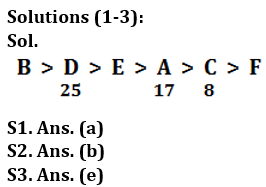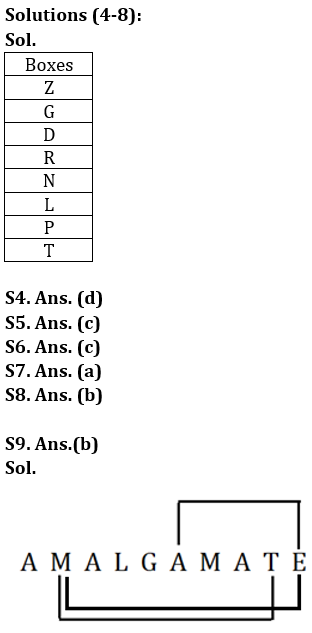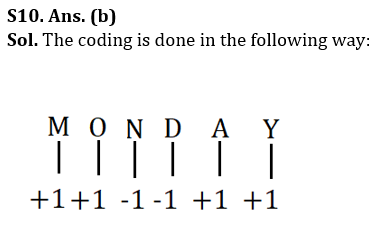# Bank of Baroda AO Reasoning Quiz 2023 – 19 March

Topic: Practice Set

Directions (1-3): Study the following information carefully and answer the questions given below-

Q1. What is the possible number of books E has read?
(a) 18
(b) 26
(c) 15
(d) 12
(e) 10

Q2. How many people read more books than E?
(a) None
(b) Two
(c) Four
(d) Five
(e) Three

Q3. Find the sum of the number of books read by A and D.
(a) 33
(b) 40
(c) 38
(d) 44
(E) 42

Directions (4-8): Study the following information carefully and answer the questions given below:
Eight boxes are placed one on top of the other. More than five boxes are placed above box P. Box D is placed above Box R and immediately below Box G. Two boxes are placed in box R and box Z. Box N is placed below box Z and above box L. Box T is placed below but not immediately below box L. At least four boxes are placed below the box R.

Q4. Which of the following is not true?
(a) Box P is placed on box T
(b) Box T is placed at the bottom
(c) Two boxes are placed below box L
(d) Box R is placed immediately above box L
(e) All are incorrect

Q5. The number of boxes placed below box G is equal to the number of boxes placed above _______.
(a) N
(b) L
(c) P
(d) R
(e) None of these

Q6. How many boxes are placed above box Z?
(a) Two
(b) Three
(c) None
(d) Five
(e) Four

Q7. Which box is placed between box N and box P?
(a) L
(b) T
(c) Z
(d) R
(e) None of these

Q8. If box P is related to box L, box N is related to box R, similarly box G is related to _________?
(a) d
(b) Z
(c) R
(d) T
(e) None of these

Q9. How many pairs of letters are there in the word ‘AMALGAMATE’, in which the middle of each letter is as many letters as the middle of the word in the English alphabet (both forward and backward)?
(a) One
(b) Three
(c) Two
(d) Four
(e) None

Q10. In a certain code language, “MONDAY” is coded as “NPMCBZ” and “SUNDAY” is coded as “TVMCBZ”, then what is the code for the word “FRIDAY”?
(a) ESHCBZ
(b) GSHCBZ
(c) FSHDBZ
(d) ESICBZ
(e) EQHCBZ

Q11. How many pairs of letters are there in the word “throughout”, each of which has as many letters as the English alphabet?
(a) Three
(b) one
(c) Two
(d) None
(e) More than three

Q12. Which of the following elements is marked with a question mark?’ should come in place of
(a) MN12
(b) OP11
(c) No. 12
(d) NM12
(e) NP11

Q13. Which of the following symbols stand for sign (\$) and sign respectively in the given expression?
Q≥M and F
Q≥E=S\$MO*D>F
(a) >, =
(b) =
(c) ≤, =
(d) >, ≤

(e) =
Q14. W>HWhich of the following will definitely NOT be true if D≤L=F>E>G is definitely true?
(a) U > D
(b) S > H
(c) F > D
(d) L>G

(e) None is true
Q15. Pointing to a man on stage, Karan said, “He is the only son of my wife’s daughter-in-law. How is the person on the platform related to Karan?
(a) Cousin
(b) Brother
(c) Son
(d) grandson

(E) NephewBank of Baroda AO Reasoning Quiz 2023 – 19 March |_60.1
S11. Ans.(a) Sol.

GH

S12. Ans.(d)

S13. Ans.(b)

S14. Ans.(c)Bank of Baroda AO Reasoning Quiz 2023 – March 19 |_80.1